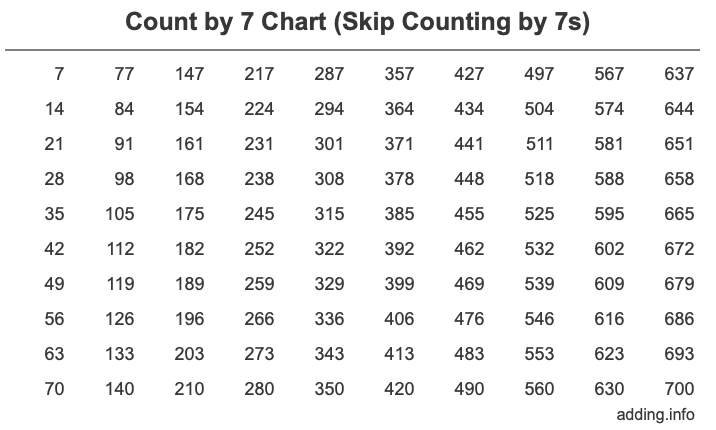Count by 7

Here we will show you how to count by 7, discuss counting by 7 patterns, and tell you why knowing how to count by 7 matters. To start off, note that Count by 7 means counting in 7s, or count by sevens, and it is also called skip counting by 7.

How to count by 7
Normally, we would count by 1 like this: 1, 2, 3, 4, etc., but when we count by 7, we count 7, 14, 21, 28, and so on.

In other words, to count in intervals of 7 or skip counting by 7, we start with 7 and then add 7 to get the next number, and then continue adding 7 to the previous number to keep counting by 7, like this:

7
7 + 7 = 14
14 + 7 = 21
21 + 7 = 28
28 + 7 = 35
...

You can of course skip count by 7 forever, so it is impossible to make a list of all numbers, but below is a Count by 7 Chart of the first 100 numbers to get you started.Looking at the chart above, you will see that the first column has the first ten numbers you get when you skip count by 7, the second column has the next ten numbers you get when you skip count by 7, and so forth.

Count by 7 Patterns
We organized the Skip Counting by 7s Chart above in 10 rows and 10 columns so you can easily identify patterns.

Skip counting always creates patterns. Figuring out these patterns may help you if want to count by 7, but don't have the Counting by 7s Chart above. Obviously, one pattern with counting by 7s is that the number increases by 7.

Furthermore, if you look at each row above, each number in the row has the same last digit (ones place). That means that every tenth number has the same last digit.

If you look down the columns, you will see that the last digit (ones place) repeats itself in blocks of 10 over and over. The pattern of the last digit when you count by 7 goes 7, 4, 1, 8, 5, 2, 9, 6, 3, 0 and 7, 4, 1, 8, 5, 2, 9, 6, 3, 0 and so on for as long as you count by 7.

Why Count by 7?
We think that understanding and learning about skip counting by 7 is important, because it teaches you how the arithmetic operations fit together. Below are some examples of what we mean.

When you count by seven, you are also creating a list of multiples of 7 that you can use in math when you need the least common multiple. 7 times n equals the nth multiple or skip count of 7.

When you skip count by 7, you are also creating a list of numbers that 7 is divisible by. On top of that, skip counting by 7 is the same as making the 7 times table.

Skip Counting
Need to skip count by another number? Enter another number for us to skip count for you.

Count by 8
Here is the next number on our list that we used to skip count.